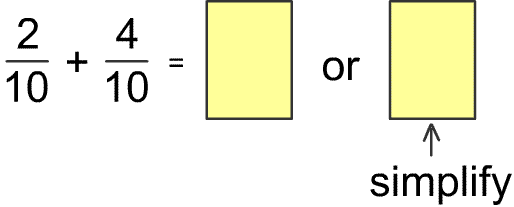Skill 26B Add Like Fractions (Same Denominator) Here we add like fractions again as in the previous skill, but also have the student "simplify" the answer. Simplify means exactly the same as "reducing to lowest terms." So if we add the problem below we get 6/10 which is put in the first yellow box. If we simplify 6/10 we get 3/5. So how did we get 3/5? To simplify an answer you find the largest number that will divide evenly into both the numerator and denominator. 2 is the largest number that divides evenly into both 6 and 10. 2 goes into 6 three times and 2 goes into 10 five time so the simplified answered is 3/5. 1. Find the sum.  Write it in the yellow box.2. Write the sum in simplest form in the second yellow box.